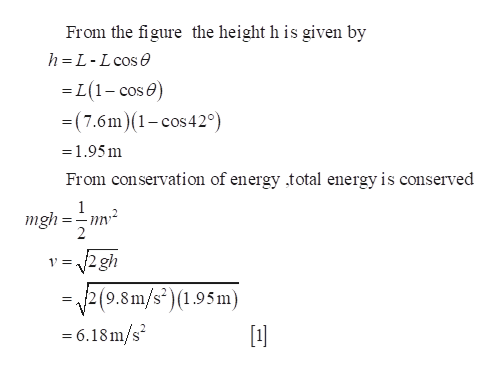Jeff of the Jungle swings on a 7.6-m vine that initially makes an angle of 42 ∘ with the vertical.If Jeff starts at rest and has a mass of 78 kg , what is the tension in the vine at the lowest point of the swing?

Question

Jeff of the Jungle swings on a 7.6-m vine that initially makes an angle of 42 ∘ with the vertical.

If Jeff starts at rest and has a mass of 78 kg , what is the tension in the vine at the lowest point of the swing?

Step 1

The below figure represents the swing of jeff of the jungle.

Step 2help_outlineImage TranscriptioncloseFrom the figure the height h is given by h= L- L cose L(1-cos e) =(7.6m)(1-cos420) - 1.95m From conservation of energy total energy is conserved 1 mgh 2 2 gh 1 2(9.8m/s)(1.95m) =6.18m/s 1] fullscreen

Want to see the full answer?

See Solution

Want to see this answer and more?

Our solutions are written by experts, many with advanced degrees, and available 24/7

See Solution
Tagged in

Physics